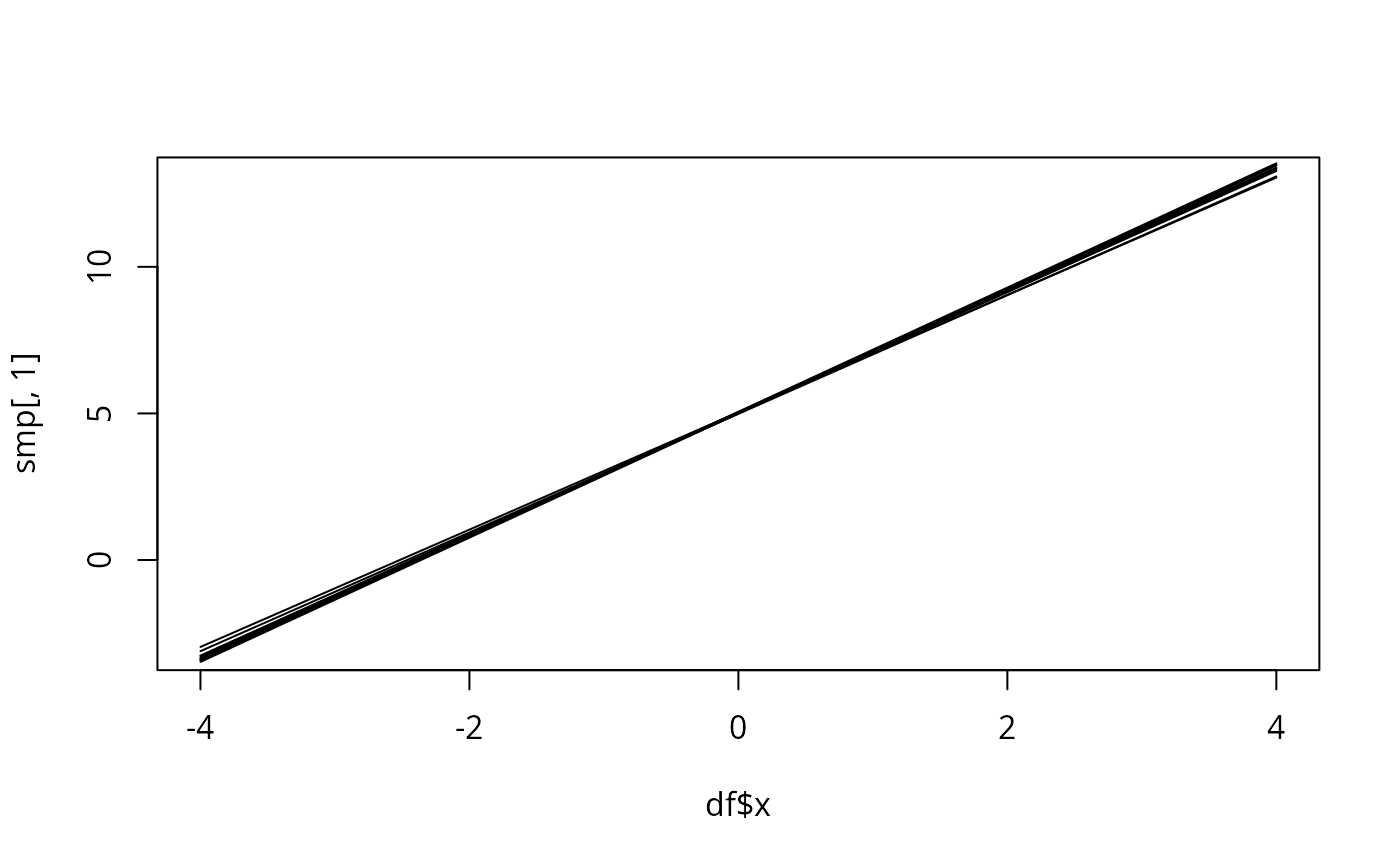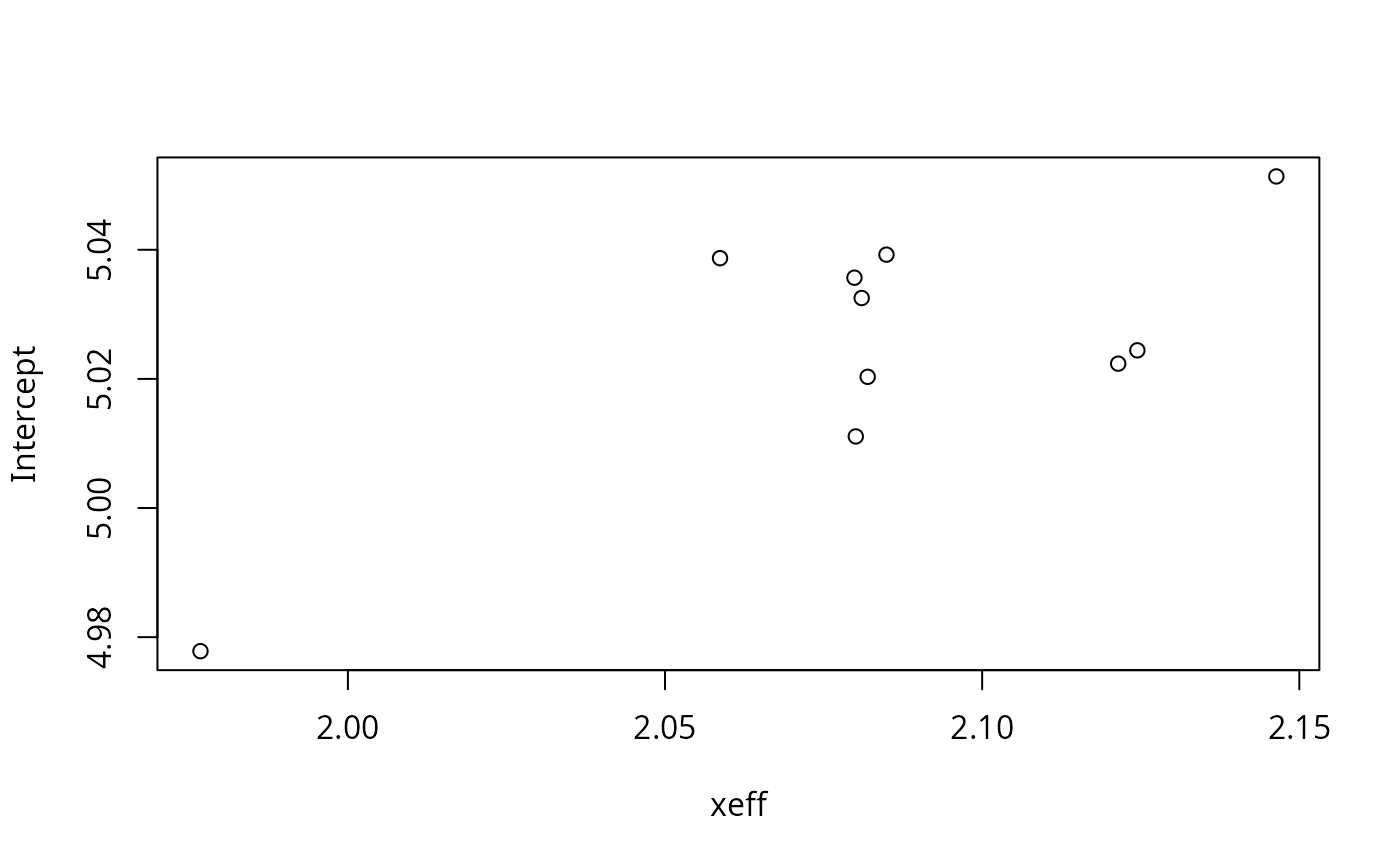Generic function for sampling for fitted models. The function invokes particular methods which depend on the class of the first argument.

Takes a fitted bru object produced by the function bru() and produces samples given a new set of values for the model covariates or the original values used for the model fit. The samples can be based on any R expression that is valid given these values/covariates and the joint posterior of the estimated random effects.

## Usage

generate(object, ...)

# S3 method for bru
generate(
object,
newdata = NULL,
formula = NULL,
n.samples = 100,
seed = 0L,
include = NULL,
exclude = NULL,
used = NULL,
...,
data = deprecated()
)

## Arguments

object

A bru object obtained by calling bru().

...

newdata

A data.frame or SpatialPointsDataFrame of covariates needed for sampling.

formula

A formula where the right hand side defines an R expression to evaluate for each generated sample. If NULL, the latent and hyperparameter states are returned as named list elements. See Details for more information.

n.samples

Integer setting the number of samples to draw in order to calculate the posterior statistics. The default, 100, is rather low but provides a quick approximate result.

seed

Random number generator seed passed on to INLA::inla.posterior.sample

Specification of desired number of threads for parallel computations. Default NULL, leaves it up to INLA. When seed != 0, overridden to "1:1"

include

Character vector of component labels that are needed by the predictor expression; Default: NULL (include all components that are not explicitly excluded) if newdata is provided, otherwise character(0).

exclude

Character vector of component labels that are not used by the predictor expression. The exclusion list is applied to the list as determined by the include parameter; Default: NULL (do not remove any components from the inclusion list)

used

Either NULL or a bru_used() object, overriding include and exclude.

data

Deprecated. Use newdata instead. sampling.

## Value

The form of the value returned by generate() depends on the data class and prediction formula. Normally, a data.frame is returned, or a list of data.frames (if the prediction formula generates a list)

List of generated samples

## Details

In addition to the component names (that give the effect of each component evaluated for the input data), the suffix _latent variable name can be used to directly access the latent state for a component, and the suffix function _eval can be used to evaluate a component at other input values than the expressions defined in the component definition itself, e.g. field_eval(cbind(x, y)) for a component that was defined with field(coordinates, ...) (see also component_eval()).

For "iid" models with mapper = bru_mapper_index(n), rnorm() is used to generate new realisations for indices greater than n.

## Examples

# \donttest{
if (bru_safe_inla(multicore = FALSE) &&
require("sn", quietly = TRUE)) {

# Generate data for a simple linear model

input.df <- data.frame(x = cos(1:10))
input.df <- within(input.df, y <- 5 + 2 * cos(1:10) + rnorm(10, mean = 0, sd = 0.1))

# Fit the model

fit <- bru(y ~ xeff(main = x, model = "linear"),
family = "gaussian", data = input.df
)
summary(fit)

# Generate samples for some predefined x

df <- data.frame(x = seq(-4, 4, by = 0.1))
smp <- generate(fit, df, ~ xeff + Intercept, n.samples = 10)

# Plot the resulting realizations

plot(df$x, smp[, 1], type = "l") for (k in 2:ncol(smp)) points(df$x, smp[, k], type = "l")

# We can also draw samples form the joint posterior

df <- data.frame(x = 1)
smp <- generate(fit, df, ~ data.frame(xeff, Intercept), n.samples = 10)
smp[]

# ... and plot them
if (require(ggplot2, quietly = TRUE)) {
plot(do.call(rbind, smp))
}
}
#>
#> Attaching package: ‘sn’
#> The following object is masked from ‘package:stats’:
#>
#>     sd# }
# \donttest{
if (bru_safe_inla(multicore = FALSE) &&
require("sn", quietly = TRUE)) {

# Generate data for a simple linear model

input.df <- data.frame(x = cos(1:10))
input.df <- within(input.df, y <- 5 + 2 * cos(1:10) + rnorm(10, mean = 0, sd = 0.1))

# Fit the model

fit <- bru(y ~ xeff(main = x, model = "linear"),
family = "gaussian", data = input.df
)
summary(fit)

# Generate samples for some predefined x

df <- data.frame(x = seq(-4, 4, by = 0.1))
smp <- generate(fit, df, ~ xeff + Intercept, n.samples = 10)

# Plot the resulting realizations

plot(df$x, smp[, 1], type = "l") for (k in 2:ncol(smp)) points(df$x, smp[, k], type = "l")

# We can also draw samples form the joint posterior

df <- data.frame(x = 1)
smp <- generate(fit, df, ~ data.frame(xeff, Intercept), n.samples = 10)
smp[]

# ... and plot them
if (require(ggplot2, quietly = TRUE)) {
plot(do.call(rbind, smp))
}
}